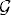# CONDITIONAL EXPECTATION

In probability theory, the conditional expectation is the expected value of a random variable given some information represented by a σ-algebra. The conditional expectation defined on the basis of a σ-algebra is again a random variable satisfying certain properties. In this chapter, we present the definition of conditional expectations based on this approach.

# 14.1 Basic Concepts and Facts

Definition 14.1 (Conditional Expectation of a Random Variable Given a σ-Algebra). Let (Ω,, P) be a probability space, and X a random variable with E(|X|) < ∞. Let G be a sub-σ-algebra of. Then a version of the conditional expectation of X given G is defined to be a random variable Y (see Theorem 14.1) such that

(a) Y is G-measurable.
(b) E(|Y|) < ∞.
(c) For every GG, we haveThe version of the conditional expectation of X givenis written as Y = E(X|) a.s.

Definition ...

Get Measure, Probability, and Mathematical Finance: A Problem-Oriented Approach now with the O’Reilly learning platform.

O’Reilly members experience live online training, plus books, videos, and digital content from nearly 200 publishers.